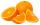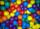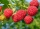# Fractions + divisibility - math problems

#### Number of problems found: 11

• Prime divisorsFind 2/3 of the ratio of the sum and the product of all prime divisors of the number 120.
• Infinite decimalImagine the infinite decimal number 0.99999999 .. ... ... ... That is a decimal and her endless serie of nines. Determine how much this number is less than the number 1. Thank you in advance.
• LCD 2The least common denominator of 2/5, 1/2, and 3/4
• Reminder and quotientThere are given the number C = 281, D = 201. Find the highest natural number S so that the C:S, D:S are with the remainder of 1,
• OrangesMother divided her three children's oranges in a ratio of 6:5:4. Two children gave 45 oranges. How many oranges were there?
• Drawing from a hatWhen drawing numbers from a hat from 1 to 35, we select random given numbers. What is the probability that the drawn numbers will be divisible by 8 and 2?
• The ballsYou have 108 red and 180 green balls. You have to be grouped into the bags so that the ratio of red to green in each bag was the same. What smallest number of balls may be in one bag?
• RatioAlena collected 7.8 kg of blueberries, 2.6 kg of blackberries, and 3.9 kg of cranberries. Express the ratio in the smallest natural numbers in this order.On the meadow grazing horses, cows, and sheep, together with less than 200. If cows were 45 times more, horses 60 times more, and sheep 35 times more than there are now, their numbers would equally. How many horses, cows, and sheep are on the meadow toget
• Reminder and quotientThere are given numbers A = 135, B = 315. Find the smallest natural number R greater than 1 so that the proportions R:A, R:B are with the remainder 1.
• DiophantusWe know little about this Greek mathematician from Alexandria, except that he lived around 3rd century A. D. Thanks to an admirer of his, who described his life through an algebraic riddle, we know at least something about his life. Diophantus's youth las

We apologize, but in this category are not a lot of examples.
Do you have an interesting mathematical word problem that you can't solve it? Submit a math problem, and we can try to solve it.

We will send a solution to your e-mail address. Solved examples are also published here. Please enter the e-mail correctly and check whether you don't have a full mailbox.

Please do not submit problems from current active competitions such as Mathematical Olympiad, correspondence seminars etc...

Need help calculate sum, simplify or multiply fractions? Try our fraction calculator. Fraction Word Problems. Divisibility - math word problems.